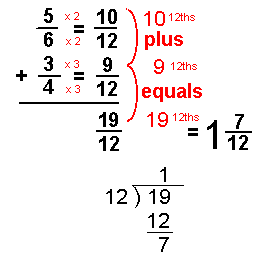# Working with Fractions1.   Write the numbers vertically:

Go to the NEXT step...

2.   Do you have a Common Denominator?
(same bottom number = same size pieces)

NO:   Continue below...

Change to equivalent fractions with a common denominator:

• Find a number divisible by BOTH denominators (such as the LCM: Least Common Multiple).
• Multiply both the numerator AND denominator of one fraction by the SAME NUMBER to get an equivalent fraction with the common denominator.
• Repeat with the other fraction.

Go to the NEXT step...

• Add the numerators   (to find the total number of pieces).
Keep the same denominator.

• Add the whole numbers (if any).

Go to the NEXT step...

4.   Is the Fraction in Lowest Terms?

NO:   Continue below...

Improper fraction:   (Top number is greater than the bottom number.)
• Divide the top BY the BOTTOM;
• Write any remainder as the numerator of a fraction using the same denominator.

Reduce the fraction:
• Divide both the numerator and the denominator by the same number.
• Repeat until the only common factor is one.

Go to the NEXT step...

SAMPLE PROBLEM #1:
 2/7 + 4/7 =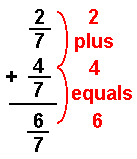SAMPLE PROBLEM #2:

 2/3 + 1/4 =

Step 1: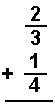Step 2: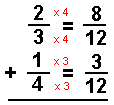Solution: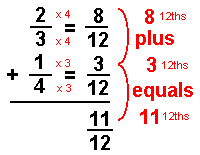SAMPLE PROBLEM #3:

 5/6 + 3/4 =

Step 1: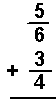Step 2: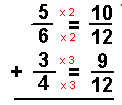Solution: